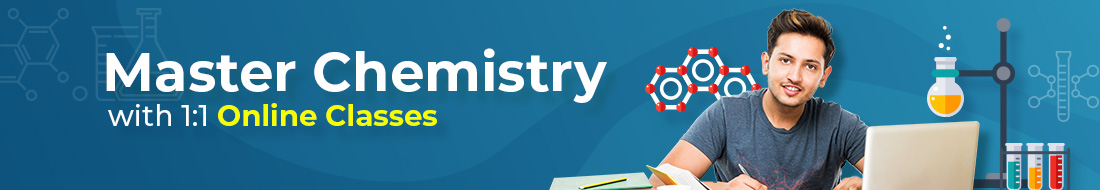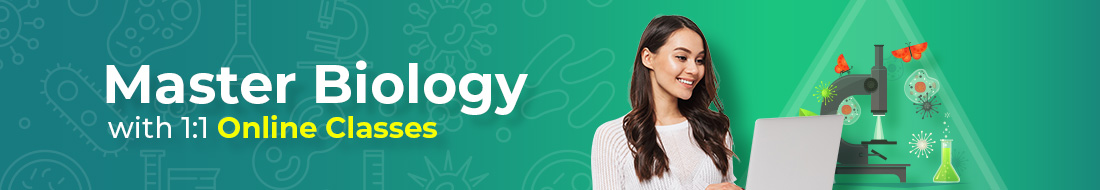#### Need Help?

Get in touch with us1-646-564-2231

# Proton Mass – Units and Properties

## Proton Mass

If you break up the particles that form the human body into smaller bits, you will find that at every step of the way, the whole equals the sum of the individual parts, especially for mass. If your body is broken down into individual fat, bones, and organs, those will combine to give the entire human being. If those are broken down further into cells, those cells would still combine to give the same mass as you. Cells can be broken down into organelles; organelles can be divided into individual molecules, which can be further broken down into atoms. These atoms can further be divided into protons, electrons, and neutrons.

A carbon atom comprises six neutrons and six protons, which is approximately 0.8% lighter than the individual particles that build it. The method by which carbon is formed is by nuclear fusion of hydrogen into helium and helium to carbon. The energy released in this process fuels most types of stars in both their red giant and normal phases. This energy actually came from the lost mass, according to Einstein’s formula E = mc2## What is a proton?

If you are wondering what is a proton, the proton definition is that it is a subatomic entity having a positive charge that is equivalent in magnitude to an electron charge unit and a rest mass equal to 1.67263 ×10-27  kg. Protons, along with neutrons, form all the atomic nuclei except hydrogen nuclei that have a single proton. The same number of protons are present in every nucleus of a given element, and it defines its atomic number and position in the periodic table. When the proton count in a nucleus is equal to the electron count orbiting around it, the atom is supposed to be electrically neutral.

### Discovery of Protons

The discovery of protons was made when the earliest investigations of atomic structures were back in history. While observing streams of ionized molecules and atoms in gaseous form from which electrons were stripped, Wilhelm Wien and J.J. Thomson identified a positive particle with a mass equal to a hydrogen atom. The ejection of hydrogen nuclei from nitrogen was shown by Ernest Rutherford in 1919 on the bombardment of alpha particles. He accepted hydrogen as an elementary particle by 1920 and named it a proton.The structural understanding of the nature of the proton within the group of subatomic particles was refined by particle-physics studies in the later years of the 20th century. It was shown that protons and neutrons are composed of smaller particles. They are classified as baryons, which refer to particles made up of three elementary matter units called quarks.

High velocities are given in particle accelerators to protons formed from ionized hydrogen. These are commonly used to study and produce nuclear reactions in the form of projectiles. Primary cosmic rays mainly contain protons. Those are also among the main products of some artificial nuclear reactions.### Mass of Proton Definition

The mass of what is a proton is the sum of the masses of its binding gluons and current quarks. It is equal to:

mp = 1.672621898(21)×10−27 KgThe mass of a proton is more than that of an electron.

### Mass of Protons in other Units

The mass of a proton can be expressed in terms of various other units, such as the mass of the proton in kg, proton mass AMU, and proton mass MeV. Below is a table that shows the value of proton mass with its corresponding units.Mass of a proton Value mass of the proton in grams 1.672621898(21)×10−24 g proton mass MeV 938.2720813(58) MeV/c2 mass of the proton in kg 1.672621898(21)×10−27 kg Mass of proton in AMU 1.007276466879(91) u

### Discovery of a Proton

In 1886, Goldstein discovered that the positive particles’ charge to mass ratio depends on the type of gas present in the discharge tube. This indicates that the charge-to-mass ratio varies in the case of different gasses. He also observed that the highest positive ray charge to mass ratio was for hydrogen gas used in the discharge tube. The main reason for this is that hydrogen atoms are the lightest and have the least mass. Therefore, its e/m ratio is the highest. The particle in the discharge tube with positive rays was called a proton, which is produced when an electron is removed from the hydrogen atom.

Hydrogen atom or H → e– (electron) + H+ (proton)Therefore, a proton is a hydrogen ion (H+).

## Properties of Protons

The particle proton is positively charged and has the physical properties as follows:Proton mass: The mass of a proton equals that of the hydrogen atom. There is one proton and one electron in a hydrogen atom. As an electron is known to have negligible mass, we can say that the mass of a hydrogen atom equals the mass of a proton. The proton mass is 1840 times the mass of an electron.

Proton mass = 1.676 × 10-27 kg= 1.676 × 10-24 g

= 1.6726219 × 10-27 kgProton charge: The proton charge is equal to and is opposite to that of an electron. Therefore, it has a positive unit charge. The charge on a proton is equal to +1.602 × 10-19 coulombs.

### How to calculate the mass of a proton?

The mass of a proton can be calculated in three ways, all of which employ different approaches to determine the atomic mass of a particle. Following are the three methods:

Calculation from theory: This method involves deriving the mass of a proton by using relativity and quantum theory. The internal structure of protons has three quarks or forces that are held together by gluons or attraction forces. According to naive norms, each quark was given about ⅓ of the proton mass. In reality, however, 95-98% of the mass of a proton does not constitute the quark mass. Rather, most of the proton mass is derived from the interaction energy among the quarks. This phrase can be best understood by recalling E = mc2.

By the molar mass of an atom: One mole of a hydrogen atom is equal to 6.022e23. Just imagine it as a measure, just like one pair equals two and one dozen equals twelve. One mole of the atom in the given hydrogen atom weighs 1.0079, while each mole of its protons weighs 1.0079 g.

Additionally, we know that the weight of a proton is equal to 1.0079 g, and one mole is equal to 6.022e23 units .If we divide the proton weight with the mole number, that is, (1.0079/ 6.022e23), we get the proton mass 1.6737e-24 g.

Note: The proton charge in an atom is balanced by an electron. Also, this experiment can be performed with any of the elements in the periodic table.

Comparison of charge/mass with electron: By this method, the bend of an atom is measured when it enters the standardized electric or magnetic fields. Additionally, the magnitude of bending indicates the mass of the proton by comparing it with the mass of the electron.

It is important to note that the notion of an experiment is the same as the induction of motion in a moving ball. Additionally, a constant mechanical force will lead to a deflection of a heavy proton definition from a straight-line movement to a small extent in every case. On the other hand, a small ball of electrons is deflected even more.

### Solved Question on Proton Mass

Question. What is the mass of the atom of a proton?

1. 1.674929 x 10-27 kg
2. 1.660540 x 10-27 kg
3. 9.109390 x 10-31 kg
4. 1.672623 x 10-27 kg

Answer. Here the correct option is C. Because options D and B are the atomic mass of an electron and neutron, respectively, and the first option (A) is the atomic mass of an atom unit.

### Conclusion

Hope the above discussion helped clear most of your doubts regarding the topic of Proton Mass and broadens your fundamental knowledge of the concept. You can solve numerical problems on this topic to increase the domain of your knowledge on this topic further.

## Frequently Asked Questions (FAQs)

### 1.Do electrons have mass?

An electron is believed to be massless if we compare it to the masses of protons and neutrons. Its mass is almost negligible. Also, we do not consider the mass of an electron while calculating an atom’s mass number.

### 2.What does the inside of a proton look like?

Every atomic nucleus has a proton which comprises three quarks held together by gluons.

### 3.What are electrons, protons, and neutrons?

Every atom has three small parts— electrons, neutrons, and protons. The neutrons and protons lie in the nucleus in the atom’s center, which has electrons around it. Protons are positively charged particles, while electrons are negatively charged.#### Solar System – What does the Solar System consist of?

As a child, you must have found the Moon and …

#### Meteorite – Definition, Classification and Components.

You look into the clear night sky, where the stars …

#### Electrical Symbols for Circuit Components.

Electrical diagram symbols and electric symbol charts created by engineers …

#### Types of Energy – What is Energy, Renewable Resources.

The ability or power to do work, such as the …

#### Blogs#### Is 34 a Good ACT Score? How Can I Improve It?

Let’s look at the average ACT score you’ll need for a great application and how you may improve it.#### How Much Do AP Tests Cost? Everything You Need to Know

Students may be eligible for both types of rewards based on their AP exam scores and the college’s policies.#### Best Online Coding Classes For Kids

Coding has become a vital piece of a kid’s education and a wonderful opportunity for them to display their creativity and ingenuity.#### With Turito Academy.

Get 7 days Free Trail Now#### With Turito Foundation.

Get a Free Demo#### Get an Expert Advice From Turito.

Get a Free Demo#### With Turito Academy.

Get 7 days Free Trail Now

#### Test PrepGet a Free Demo